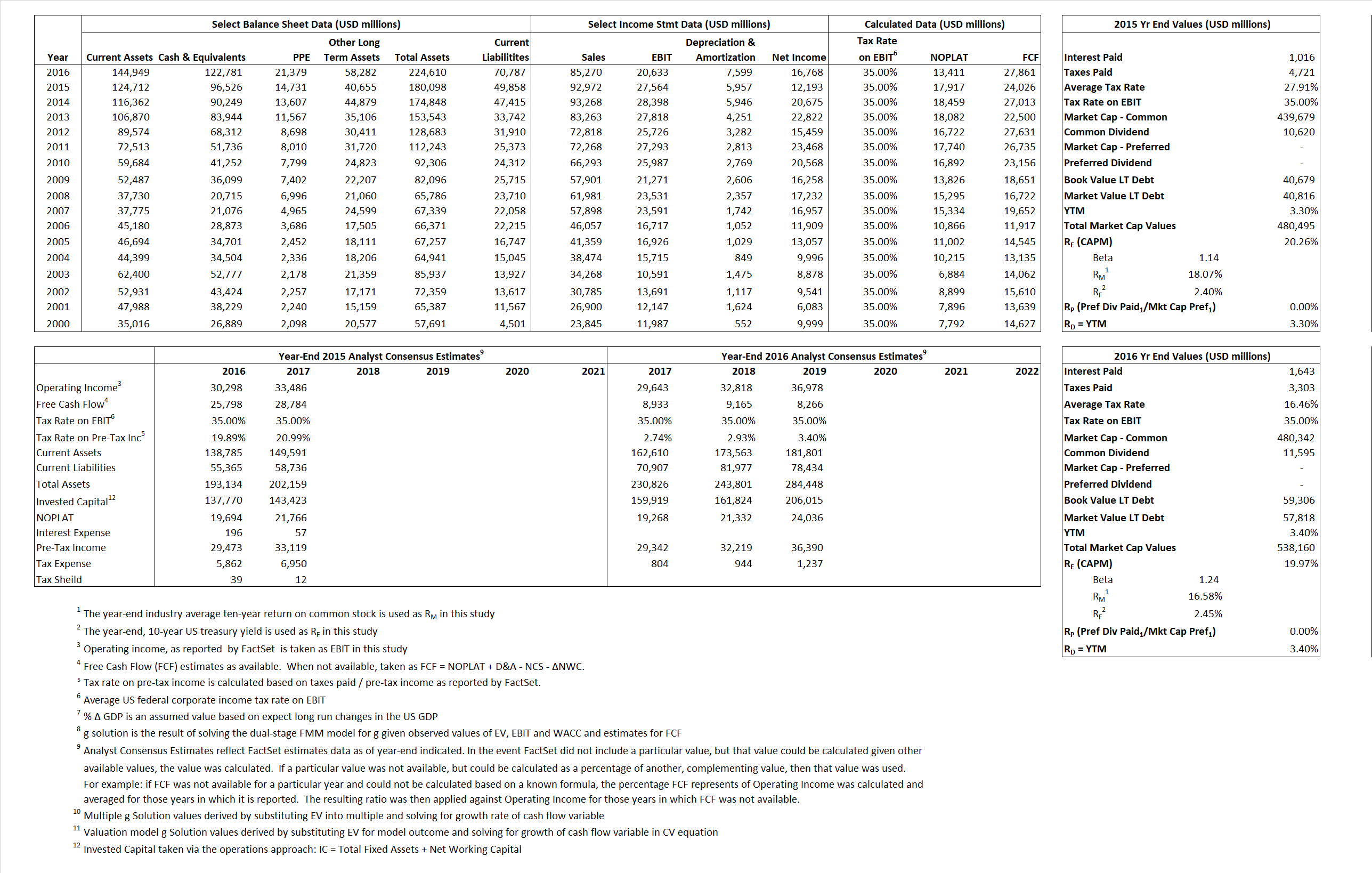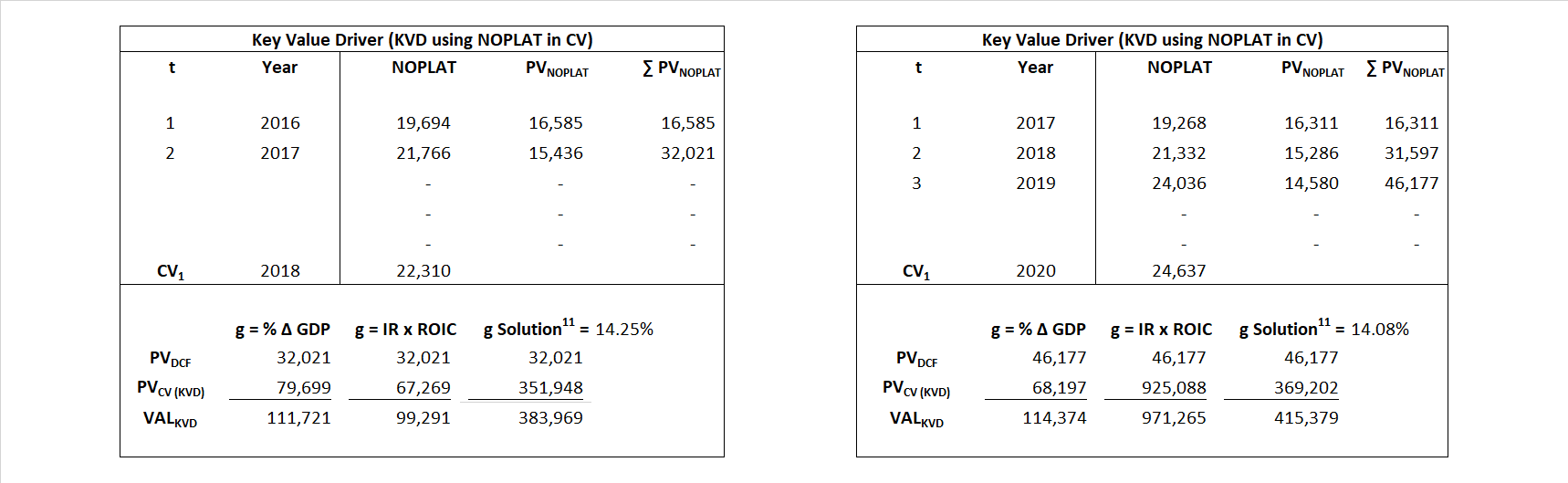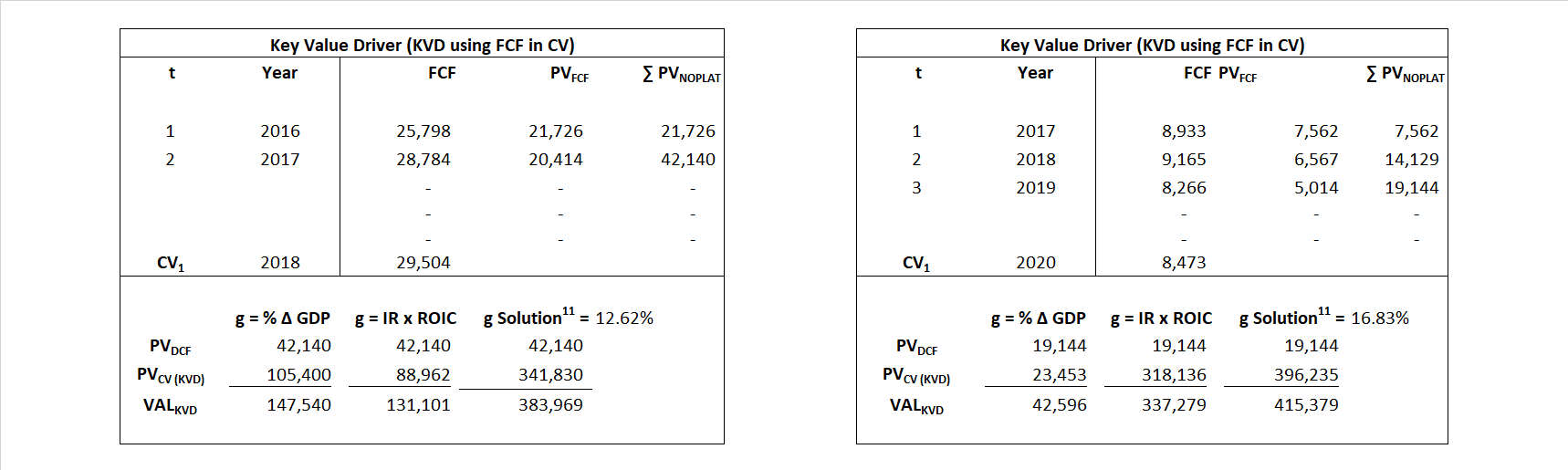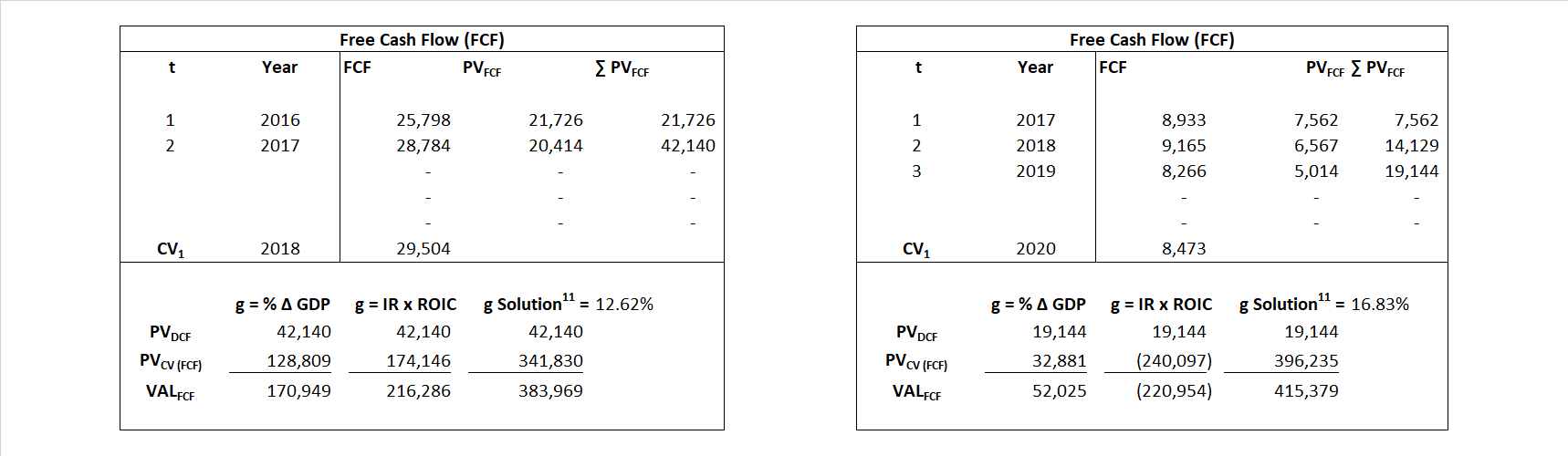# Microsoft

## Analyst Listing

The following analysts provide coverage for the subject firm as of May 2016:

 Broker Analyst Analyst Email Stifel Nicolaus Brad R. Reback rebackb@stifel.com Pacific Crest Securities-KBCM Brendan Barnicle bbarnicle@pacific-crest.com Atlantic Equities Christopher Hickey c.hickey@atlantic-equities.com BGC Financial, L.P. Colin W. Gillis cgillis@bgcpartners.com Nomura Research Frederick Grieb frederick.grieb@nomura.com Cowen & Company Gregg Moskowitz gregg.moskowitz@cowen.com Griffin Securities Jay Vleeschhouwer jvleeschhouwer@griffinsecurities.com Jefferies John DiFucci jdifucci@jefferies.com Deutsche Bank Research Karl Keirstead karl.keirstead@db.com Daiwa Securities Co. Ltd. Kazuya Nishimura kazuya.nishimura@us.daiwacm.com BMO Capital Markets Keith Bachman keith.bachman@bmo.com Evercore ISI Kirk Materne kirk.materne@evercoreisi.com Bernstein Research Mark L. Moerdler mark.moerdler@bernstein.com Raymond James Michael Turits michael.turits@raymondjames.com Credit Suisse Philip Winslow philip.winslow@credit-suisse.com Wunderlich Securities Rob Breza robbreza@wundernet.com RBC Capital Markets Ross MacMillan ross.macMillan@rbccm.com Cross Research Shannon Cross shannon@crossresearch.com FBN Securities Shebly Seyrafi sseyrafi@fbnsecurities.com Hilliard Lyons Stephen Turner sturner@hilliard.com Oppenheimer Timothy Horan tim.horan@opco.com

## Primary Input Data## Derived Input Data

### Equational Form

Net Operating Profit Less Adjusted Taxes NOPLAT  17,917   13,411$NOPLAT\, =\, EBIT\, x\, (1 \,-\, Avg \,\,Tax\,\, Rate\,\, on\,\, EBIT)$
Free Cash Flow FCF  24,026  27,861$FCF\,=NOPLAT\,+\,Non-Cash\,Expenses-\Delta NWC\,-\,NCS$
Tax Shield TS  284  270$TS\,=\,Interest\,\,Paid\,\,x\,\, Avg \,\,Tax\,\,Rate\,\, on\,\, Pre-Tax\,\, Income$
Invested Capital IC  130,240  153,823$IC\,=\,Fixed\,\,Operating\,\,Assets\,\,+\,\,Net\,\, Working\,\, Capital$
Return on Invested Capital ROIC 13.76% 8.72%$ROIC\,=\,\frac { NOPLAT }{ IC }$
Net Investment NetInv  8,764  31,182$NetInv\,=\,{ {IC}_{1}}-{{IC}_{0}}+Depreciation$
Investment Rate IR 48.92% 232.50%$IR\,=\,\frac {NetInv}{NOPLAT}$
Weighted Average Cost of Capital WACCMarket 18.74% 18.13%$WACC\,=\,\frac { E }{ V } { R }_{ E }\,+\,\frac { P }{ V } { R }_{ P }\,+\,\frac { D }{ V } { R }_{ D }\left( 1- Avg\,\, Tax\,\,Rate\,\,on\,\,Pre-Tax\,\,Income \right)$
WACCBook  9.92% 9.27%
Enterprise value EVMarket  383,969  415,379$EV\,=\,Market\,\,Cap\,\,Equity\,+\,\,Long\,\,Term\,\,Debt\,-\,Cash$
EVBook  357,577  416,867
EV/EBIT Multiple$\frac{EV_{Market}}{EBIT}$  13.93  20.13$EV/EBIT\,=\,\frac { EV}{ EBIT}$
Long-Run Growth g = IR x ROIC
6.73% 20.27% Long-run growth rates of the income variable  are used in the Continuing Value portion of the valuation models.
g = %$\Delta$ GDP   2.50%   2.50%

## Valuation Model Outcomes

The outcomes presented in this study are the result of original input data, derived data, and synthesized inputs and, depending on the equational form of any particular valuation model, may result in irrelevant or implausible results.  For example, in the event WACC < g, the value of this term, often found in the denominator of an equation’s continuation value term, will be expressly negative and may result in a negative overall valuation for the firm.  In the event of a WACC < g relation, the model form as applied to the subject firm offers an irrelevant outcome.

### Equational form

Key Value Driver (NOPLAT) KVD (NOPLAT)${ Value }_{ DCF/KVD }=\sum { \frac { NOPLAT_{ t } }{ { \left( 1+WACC \right) }^{ t } } +\frac { \frac { { NOPLAT }_{ 1 }\left( 1-\frac { g }{ ROIC } \right) }{ WACC-g } }{ { \left( 1+WACC \right) }^{ t } } }$Key Value Driver (FCF) KVD (FCF)${ Value }_{ DCF/KVD }=\sum { \frac { FCF_{ t } }{ { \left( 1+WACC \right) }^{ t } } +\frac { \frac { { NOPLAT }_{ 1 }\left( 1-\frac { g }{ ROIC } \right) }{ WACC-g } }{ { \left( 1+WACC \right) }^{ t } } }$Free Cash Flow FCF${ Value }_{ DCF/FCF }=\sum { \frac { FCF_{ t } }{ { \left( 1+WACC \right) }^{ t } } +\frac { \frac { { FCF }_{ 1 }}{ WACC-g } }{ { \left( 1+WACC \right) }^{ t } } }$Economic Profit ECON π${ Value }_{ { ECON\pi } }= I{ C }_{ 0 }+\sum { \frac { { IC }_{ t-1 }(ROI{ C }_{t}-WAC{C}_{t}) }{ { \left( 1+WACC \right) }^{ t } }+ \frac {\frac { I{C}_{0}\ x\ (ROI{C}_{1}\ -\ WAC{C}_{1}) }{ WACC-g } }{ { \left( 1+WACC \right) }^{ t } } }$Adjusted Present Value APV${ Value }_{ APV }=\sum { \frac { FCF_{ t } }{ { \left( 1+{ k }_{ u } \right) }^{ t } } +\frac { \frac { { FCF }_{ 1 }}{ { k }_{ u }-g } }{ { \left( 1+{ k }_{ u } \right) }^{ t } } } +\sum { \frac { { TS }_{ t } }{ { \left( 1+{ k }_{ tax } \right) }^{ t } } +\frac { \frac { { TS }_{ 1 }}{ { k }_{ tax }-g } }{ { \left( 1+{ k }_{ tax } \right) }^{ t } } }$Forward Market Multiple FMM${ Value }_{ DCF/FMM}=\sum { \frac { FCF_{ t } }{ { \left( 1+WACC \right) }^{ t } } +\frac { { EBIT }_{ 1 }\,{x}\,{FMM}}{ { \left( 1+WACC \right) }^{ t } } }{\,\,\,; \,\,FMM\,=\,\frac{{EV}_{t=0}}{{EBIT}_{t=0}}}$